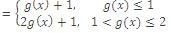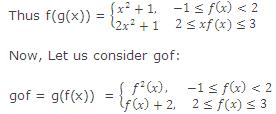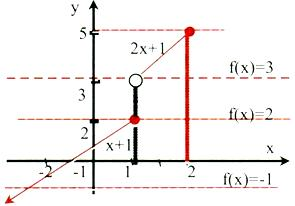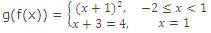# Composite Functions

Problem of finding out fog and gof can also be handled using graphical methods

f(g(x))Here g(x) becomes the variable that means we would draw the graph of g(x). It is clear that g(x) < 1 ∀ x ε [-1, 1] and 1 < g(x) < 2 ∀ x ε (1, √2]In this case f(x) becomes the variable and we will draw the graph of f(x). From the graph we observe that -1 < f(x) < 2 ∀ x ε [-2, 1) and f(x) = x + 1.

2 < f(x)) < 3 => x = 1 and f(x) = x + 1.i.e. g(f(x)) = (x + 1)2, -2 < x < 1

IIT JEE study material is available online free of cost at askIITians.com. Study Physics, Chemistry and Mathematics at askIITians website and be a winner. We offer numerous live online courses as well for live online IIT JEE preparation - you do not need to travel anywhere any longer - just sit at your home and study for IIT JEE live online with askIITians.com.

To read more, Buy study materials of Set Relations and Functions comprising study notes, revision notes, video lectures, previous year solved questions etc. Also browse for more study materials on Mathematics here.